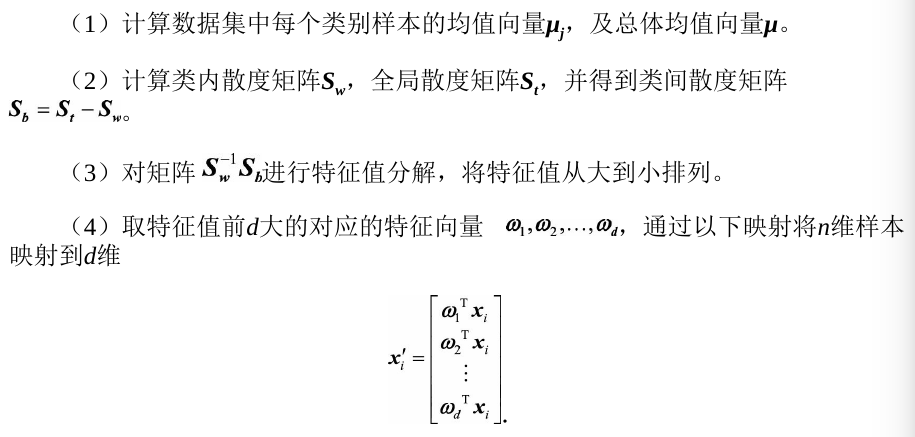# 降维算法

Posted by MaggicQ on January 9, 2019

# 降维算法

## 主成分分析

​ 主成分分析（Principal components analysis，以下简称PCA）是最重要的降维方法之一。在数据压缩消除冗余和数据噪音消除等领域都有广泛的应用。

​ PCA顾名思义，就是找出数据里最主要的方面，用数据里最主要的方面来代替原始数据。具体的，假如我们的数据集是$n$维的，共有$m$个数据$(x^{(1)},x^{(2)},…,x^{(m)})$。我们希望将这$m$个数据的维度从$n$维降到$n’$维，希望这$m$个$n’$维的数据集尽可能的代表原始数据集。我们知道数据从$n$维降到$n’$维肯定会有损失，但是我们希望损失尽可能的小。那么如何让这$n’$维的数据尽可能表示原来的数据呢？

​ 降维实际上可以看成是将$n$维空间内的样本点投射到一个超平面上，通常来说要使得投射过程中信息的损失尽可能小，这个超平面应该满足：

• 最近重构性，即样本点到这个超平面($n’$维大小的空间内的超平面)的距离足够近
• 最大可分性，即样本点在这个超平面上的投影能尽可能的分开

• 计算出整个训练集上，利用原样本点$x_i$与基于投影重构的样本点$\hat{x_i}$之间的距离
• 最小化$d$即可，详细的过程可以参考西瓜书，因为公式较多就不写出来了。

### 基于最大可分性的推导

​ 假设$m$个$n$维数据$X_{n \times m} = (x_1, x_2,…,x_m)$都已经进行了中心化，即$\sum\limits_{i=1}^{m}x_i = 0$。经过投影变换后得到的新的坐标系为$\{w_1,w_2,...,w_d\}$， 其中$w_i$是标准正交基向量，$\| w_i \|_2 = 1, w_i^Tw_j = 0(i \neq j)$，样本点$x_i$在新空间中超平面上的投影是$W^Tx_i$ ，若所有样本点的投影尽可能分开，则应该使投影后样本点的方差最大化。投影后样本点的方差为$\sum_{i}W^Tx_ix_i^TW$（注意：数据已经进行了中心化），于是优化目标可以写成：

​ 对上式使用拉格朗日乘子法：

​ 接下来只需要最大化$J(W)$即可，由于

​ 所以根据式(1)对$W$求导后得到：

​ 到这里，我们只需要对协方差矩阵$XX^T$进行特征值分解，将求得的特征值排序：$\lambda_1 \geq \lambda_2 \geq … \geq \lambda_d$，再取前$d’$个特征值对应的特征向量构成$W = (w_1, w_2, …., w_{d’})$，这就是出成分分析的解。

1. 对所有样本进行中心化：$x_i \leftarrow x_i - \frac{1}{m}\sum_{i=1}^m x_i$

2. 计算样本的协方差矩阵

3. 对协方差矩阵进行特征值分解

4. 取最大的$d’$个特征值所对应的特征向量$w_1, w_2 , … w_{d’}$作为投影矩阵$W$的列向量

### 核化线性降维

​ 线性降维方法假设从高维空间到低维空间的函数映射是线性的，然而在不少的现实任务中，可能需要非线性映射才能找到恰当的低维嵌入。这个时候就需要非线性降维。非线性降维的一种常用方法，是基于核技巧对线性降维方法进行“核化”。

​ 在主成分分析中，使用了核函数的主成分分析一般称之为核主成分分析(Kernelized PCA, 以下简称KPCA。假设高维空间的数据是由$n$维空间的数据通过映射$\phi$产生。

​ 则对于$n$维空间的特征分解：

​ 映射为：

​ 通过在高维空间进行协方差矩阵的特征值分解，然后用和PCA一样的方法进行降维。一般来说，映射$\phi$不用显式的计算，而是在需要计算的时候通过核函数完成。由于KPCA需要核函数的运算，因此它的计算量要比PCA大很多。

## 线性判别分析

​ 线性判别分析(Linear Discriminant Analysis,LDA)是一种有监督学习算法，同时经常被用来对数据进行降维。它是Ronald Fisher在1936年发明的，有些资料上也称之为Fisher LDA(Fisher’s Linear Discriminant Analysis)。LDA是目前机器学习、数据挖掘领域中经典且热门的一种算法。

​ 相比于PCA,LDA可以作为一种有监督的降维算法。在PCA中，算法没有考虑数据的标签(类别)，只是把原数据映射到一些方差比较大的方向上而已。

### 数学原理

LDA的中心思想——最大化类间距离和最小化类内距离。

​ LDA首先是为了分类服务的，因此只要找到一个投影方向$w$，使得投影后的样本尽可能按照原始类别分开。首先考虑简单的二分类问题，假设存在$C_1, C_2$两个类别的样本，两类的均值分别为$\mu_{1}=\frac{1}{N_{1}} \sum_{x \in C_{1}} x, \quad \mu_{2}=\frac{1}{N_{2}} \sum_{x \in C_{2}} x$，我们希望投影之后两类的距离尽可能大，距离表示为：

​ 其中$\widetilde{\boldsymbol{\mu}}_{1}, \widetilde{\boldsymbol{\mu}}_{2}$表示两类的中心在$w$方向上的投影向量，$\widetilde{\boldsymbol{\mu}}_{1}=\boldsymbol{\omega}^{\mathrm{T}} \boldsymbol{\mu}_{1}, \quad \widetilde{\boldsymbol{\mu}}_{2}=\boldsymbol{\omega}^{\mathrm{T}} \boldsymbol{\mu}_{2} ，$　因此需要优化的问题为：

​ 容易发现，当$w$方向与$\mu_1 - \mu_2$方向一致的时候，该距离达到最大值。除了希望投影之后两类的距离尽可能大，我们还希望同时优化类内方差，使其尽可能小。我们将整个数据集的类内方差定义为各个类分别的方差之和，经目标函数定义为类间距离和类内距离的比值：

​ 其中$w$为单位向量，$D_1, D_2$分别表示两类投影后的方差：

​ 因此$J(w)$可以写成

​ 定义类间散度矩阵$S_{B}=\left(\mu_{1}-\mu_{2}\right)\left(\mu_{1}-\mu_{2}\right)^{\mathrm{T}}$，类内散度矩阵$S_{w}=\sum_{x \in C_{i}}\left(x-\mu_{i}\right)\left(x-\mu_{i}\right)^{\mathrm{T}}$，则$J(w)$可以写成

​ 为了最大化$J(w)$，只需要对$w$求偏导，并令导数等于０：

​ 于是就有：

​ 由于在二分类问题中，$w$的维度是$2 \times 1$，$S_B$以及$S_w$的维度都是$2 \times 2$，因此$w^T S_ww$和$w^TS_Bw$是两个数，令：

​ 从这里我们可以看出,我们最大化的目标对应了一个矩阵的特征值，于是LDA降维变成了一个求矩阵特征向量的问题。$J(w)$就对应了矩阵 $S_w^{−1}S_B$最大的特征值，而投影方向就是这个特征值对应的特征向量。

​也就是说，我们只需要求样本的均值和类内方差,就可以马上得出最佳的投影方向ω。这便是Fisher在1936年提出的线性判别分析。

​下面把LDA推广到多分类的情况，假设有$N$个类别，并需要最终将特征降维到$d$维。因此，我们要找到一个$d$维投影超平面$W = {w_1, w_2, …, w_d}$，使得投影后的样本点满足 LDA的目标：最大化类间距离和最小化类内距离。

​ 在二分类中提到的类间散度矩阵在类别增加到N的时候仍满足定义，而类间散度矩阵$S_b$则无法按照原始定义。为此，我们可以定义一个新的矩阵$S_t$，来表示全局整体的散度，称为全局散度矩阵：

​ 把全局散度定义为类内散度以及类间散度之和即$S_t = S_b + S_w$，那么类间散度矩阵可以表示为：

​ 具体的推导过程如下：​其中，$m_i$是第$i$个类别中的样本个数，$N$是总的类别个数，$u$表示全部样本的中心。从上式可以看出来，类间散度表示的就是每个类别中心到全局中心的一种加权距离。我们最大化类间散度实际上优化的是每个类别的中心经过投影后离全局中心的投影足够远。

​ 其中$W$是要求解的投影超平面，由二分类中的结论可知，最大化$J(W)$对应了以下广义特征值求解的问题：

​ 求解最佳投影平面$\boldsymbol{W}=\left\{\boldsymbol{\omega}_{1}, \boldsymbol{\omega}_{2}, \ldots, \boldsymbol{\omega}_{d}\right\}$即求解$S_w^{-1}S_b$矩阵特征值前$d$大对应的特征向量组成的矩阵。这就将原始的特征空间投影到了新的d维空间中。至此我们得到了与PCA步骤类似,但具有多个类别标签高维数据的LDA求解方法：## PCA与LDA的比较

​ 结果相同，对数据进行降维。但是原理不同，在选择进行投影的超平面的时候，PCA选择的是投影后数据方差最大的方向。由于它是无监督的，因此PCA假设方差越大，信息量越多，用主成分来表示原始数据可以去除冗余的维度，达到降维。而LDA选择的是投影后类内方差小、类间方差大的方向。其用到了类别标签信息，为了找到数据中具有判别性的维度，使得原始数据在这些方向上投影后，不同类别尽可能区分开。

​ Fisher LDA相比PCA更善于对有类别信息的数据进行降维处理，但它对数据的分布做了一些很强的假设，例如,每个类数据都是高斯分布、各个类的协方差相等。尽管这些假设在实际中并不一定完全满足，但LDA已被证明是非常有效的一种降维方法。主要是因为线性模型对于噪声的鲁棒性比较好，但由于模型简单,表达能力有一定局限性，我们可以通过引入核函数扩展LDA方法以处理分布较为复杂的数据。

​ 从应用的角度,我们可以掌握一个基本的原则——对无监督的任务使用PCA进行降维,对有监督的则应用LDA。